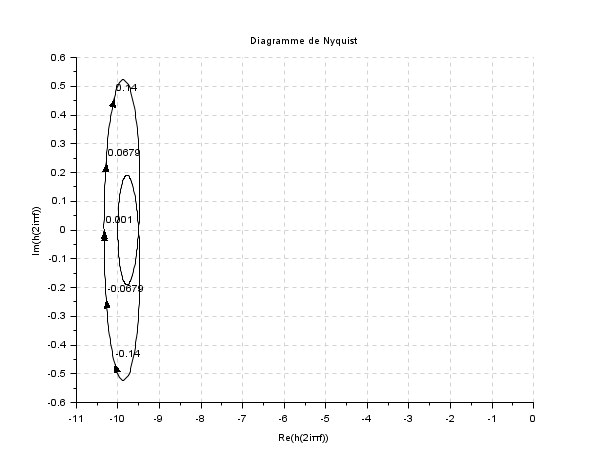Scilab Home page | Wiki | Bug tracker | Forge | Mailing list archives | ATOMS | File exchange
Change language to: English - Português - 日本語 - Русский

Please note that the recommended version of Scilab is 6.0.2. This page might be outdated.
See the recommended documentation of this function

# g_margin

gain margin and associated crossover frequency

### Syntax

```gm=g_margin(h)
[gm,fr]=g_margin(h)```

### Arguments

h

a SISO linear system (see :syslin).

gm

a number, the gain margin (in dB) if any of `Inf`

fr

a number, the associated frequency in hertz, or an empty matrix if the gain margin does not exist.

### Description

Given a SISO linear system in continuous or discrete time, `g_margin` returns `gm`, the gain margin in dB of `h` and `fr`, the achieved corresponding frequency in Hz.

The gain margin, if it exists, is the minimal value of the system gain at points where the nyquist plot crosses the negative real axis. In other words the gain margin is `20*log10(1/g)` where `g` is the open loop gain of `h` when the frequency response phase of `h` equals -180°

The algorithm uses polynomial root finder to solve the equations:

h(s)=h(-s)

for the continuous time case.

h(z)=h(1/z)

for the discrete time case.

### Examples

```h=syslin('c',-1+%s,3+2*%s+%s^2) //continuous time case
[g,fr]=g_margin(h)
[g,fr]=g_margin(h-10)
nyquist(h-10)``````h = syslin(0.1,0.04798*%z+0.0464,%z^2-1.81*%z+0.9048);//discrete time case
[g ,fr]=g_margin(h);
show_margins(h)```• p_margin — phase margin and associated crossover frequency
• show_margins — display gain and phase margin and associated crossover frequencies
• repfreq — frequency response
• black — Black-Nichols diagram of a linear dynamical system
• bode — Bode plot
• nicholschart — Nichols chart
• nyquist — nyquist plot